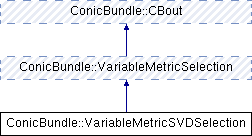ConicBundle
ConicBundle::VariableMetricSVDSelection Class Reference

general implementation of a VariableMetricSelection routine to form and add variable metric information to a BundleProxObject generically mainly from the aggregate and a collection of minorants given by a MinorantBundle More...

#include <VariableMetricSVDSelection.hxx>

Inheritance diagram for ConicBundle::VariableMetricSVDSelection:Public Member Functions

~VariableMetricSVDSelection ()
destructor

VariableMetricSVDSelection (CBout *cb=0, int cbincr=-1)
default constructor

VariableMetricSVDSelection (CH_Matrix_Classes::Integer in_n_latest_minorants, CH_Matrix_Classes::Integer in_selection_method, CH_Matrix_Classes::Real in_oldfactor=0., CBout *cb=0, int cbincr=-1)
constructor for specifying values for n_latest_minorants and selection_method

void get_UVlambda (CH_Matrix_Classes::Matrix &U, CH_Matrix_Classes::Matrix &V, CH_Matrix_Classes::Matrix &lam, CH_Matrix_Classes::Matrix &cand)
for current ongoing experiments with variable metric routines

CH_Matrix_Classes::Integer get_n_latest_minorants () const
returns n_latest_minorants

void set_n_latest_minorants (CH_Matrix_Classes::Integer nlm)
sets n_latest_minorants

CH_Matrix_Classes::Integer get_selection_method () const
returns selection_method

void set_selection_method (CH_Matrix_Classes::Integer sm)
sets selection_method

int add_variable_metric (VariableMetric &H, CH_Matrix_Classes::Integer y_id, const CH_Matrix_Classes::Matrix &y, bool descent_step, CH_Matrix_Classes::Real weightu, CH_Matrix_Classes::Real model_maxviol, const CH_Matrix_Classes::Indexmatrix *indices, VariableMetricBundleData &bundle_data)

VariableMetricSelectionclone_VariableMetricSelection ()
clone: the values are only preserved for those contained in the constructor: n_latest_minorants, selection_method and oldfactorPublic Member Functions inherited from ConicBundle::VariableMetricSelection
VariableMetricSelection (CBout *cb=0, int cbincr=-1)
constructor for passing on ouptut information

virtual ~VariableMetricSelection ()
virtual destructorPublic Member Functions inherited from ConicBundle::CBout
virtual void set_out (std::ostream *out=0, int print_level=1)
Specifies the output level (out==NULL: no output at all, out!=NULL and level=0: errors and warnings, level>0 increasingly detailed information) More...

virtual void set_cbout (const CBout *cb, int incr=-1)
Specifies the output level relative to the given CBout class. More...

void clear_cbout ()
reset to default settings (out=0,print_level=1)

CBout (const CBout *cb=0, int incr=-1)
calls set_cbout

CBout (std::ostream *outp, int pl=1)
initialize correspondingly

CBout (const CBout &cb, int incr=0)
copy constructor

virtual bool cb_out (int pl=-1) const
Returns true if out!=0 and (pl<print_level), pl<0 should be used for WARNINGS and ERRORS only, pl==0 for usual output.

std::ostream & get_out () const
If cb_out() returned true, this returns the output stream, but it will abort if called with out==0.

std::ostream * get_out_ptr () const
returns the pointer to the output stream

int get_print_level () const
returns the print_level

virtual int mfile_data (std::ostream &out) const
writes problem data to the given outstream

Private Member Functions

int vecH_by_aggregate (CH_Matrix_Classes::Matrix &vecH, CH_Matrix_Classes::Real &relprec, const MinorantBundle &bundle, const MinorantPointer &aggregate, CH_Matrix_Classes::Real function_factor, CH_Matrix_Classes::Integer y_id, const CH_Matrix_Classes::Matrix &y, CH_Matrix_Classes::Real weightu, CH_Matrix_Classes::Real violation_eps)
scaling heuristic based on collected minorants relative to the aggregate alone

int vecH_by_model (CH_Matrix_Classes::Matrix &vecH, CH_Matrix_Classes::Real &relprec, const MinorantBundle &bundle, const MinorantBundle &model, const MinorantPointer &aggregate, CH_Matrix_Classes::Real function_factor, CH_Matrix_Classes::Integer y_id, const CH_Matrix_Classes::Matrix &y, CH_Matrix_Classes::Real weightu, CH_Matrix_Classes::Real violation_eps)
scaling heuristic based on collected minorants relative to aggregate and model

int vecH_orthogonal_to_model (CH_Matrix_Classes::Matrix &vecH, CH_Matrix_Classes::Real &relprec, const MinorantBundle &bundle, const MinorantBundle &model, const CH_Matrix_Classes::Matrix &modelcoeff, const MinorantPointer &aggregate, CH_Matrix_Classes::Real function_factor, CH_Matrix_Classes::Integer y_id, const CH_Matrix_Classes::Matrix &y, CH_Matrix_Classes::Real weightu, CH_Matrix_Classes::Real violation_eps)
scaling heuristic based on collected minorants relative to aggregate and orthogonalized to model

int vecH_orthogonal_to_modelSVD (CH_Matrix_Classes::Matrix &vecH, CH_Matrix_Classes::Real &relprec, const MinorantBundle &bundle, const MinorantBundle &model, const CH_Matrix_Classes::Matrix &modelcoeff, const MinorantPointer &aggregate, CH_Matrix_Classes::Real function_factor, CH_Matrix_Classes::Integer y_id, const CH_Matrix_Classes::Matrix &y, CH_Matrix_Classes::Real weightu, CH_Matrix_Classes::Real violation_eps)
scaling heuristic based on collected minorants relative to aggregate and orthogonalized to model

int vecH_weighted_SVD (CH_Matrix_Classes::Matrix &vecH, CH_Matrix_Classes::Real &relprec, const MinorantBundle &bundle, const MinorantBundle &model, const CH_Matrix_Classes::Matrix &modelcoeff, const MinorantPointer &aggregate, CH_Matrix_Classes::Real function_factor, CH_Matrix_Classes::Integer y_id, const CH_Matrix_Classes::Matrix &y, CH_Matrix_Classes::Real weightu, CH_Matrix_Classes::Real violation_eps)
scaling heuristic based weighted sum of collected minorants relative to active subgradients and orthogonalized to model

int vecH_weighted_SVDs (CH_Matrix_Classes::Matrix &vecH, CH_Matrix_Classes::Real &relprec, const MinorantBundle &bundle, const MinorantBundle &model, const CH_Matrix_Classes::Matrix &modelcoeff, const MinorantPointer &aggregate, CH_Matrix_Classes::Real function_factor, CH_Matrix_Classes::Integer y_id, const CH_Matrix_Classes::Matrix &y, CH_Matrix_Classes::Real weightu, CH_Matrix_Classes::Real violation_eps)
scaling heuristic based weighted sum of SVD Hessians collected via minorants relative to active subgradients

Private Attributes

CH_Matrix_Classes::Integer n_latest_minorants
a positive number means dynamic scaling should be used and suggests to store and use this number of the most recent minorants for this; if <=0, no metric information will be added

CH_Matrix_Classes::Integer selection_method
specifies which of the current experimental routines should be used for computing the metric

CH_Matrix_Classes::Real oldfactor
for values of oldfactor between 0. and 1. the SVD is taken of (1-oldfactor)*vecH*vecH'+oldfactor*oldvecH*oldvcH'; for values <=0 there is no old contribution, for values >=1 oldvecH is reused

CH_Matrix_Classes::Matrix Vspace
experimental data that tries to collect the current Vspace by an orthonormal basis (only some routines attempt this)

CH_Matrix_Classes::Matrix Uspace
experimental data that tries to collect the current Uspace information by an orthonormal basis (only some routines attempt this)

CH_Matrix_Classes::Matrix lambda
experimental data that tries to identify the curvature (Hessian eigenvalues) in Uspace directions (only some routines attempt this)

CH_Matrix_Classes::Matrix candNewton
experimental candidate that results from an approximate Newton step computaiton based on Vspace, Uspace and lambda (only some routines compute this)

Detailed Description

general implementation of a VariableMetricSelection routine to form and add variable metric information to a BundleProxObject generically mainly from the aggregate and a collection of minorants given by a MinorantBundle

the class currently also includes a number of experimental routines for computing suitable low rank metric information from the latest minorant data

The documentation for this class was generated from the following file: